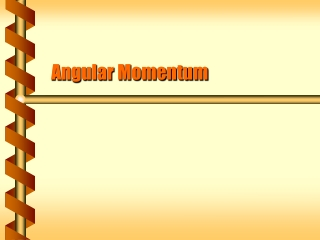DownloadDownload PresentationAngular Momentum

# Angular Momentum

Télécharger la présentation## Angular Momentum

- - - - - - - - - - - - - - - - - - - - - - - - - - - E N D - - - - - - - - - - - - - - - - - - - - - - - - - - -
##### Presentation Transcript

1. Angular Momentum

2. Extending Momentum • In linear motion we began with mass and acceleration, and extended it to momentum. • F = ma • p = mv • To continue the analysis of rotational motion, we must also extend the idea of momentum. p r

3. Applying Torque • If torque is from force, and angular momentum is from momentum, … • An external torque changes angular momentum. L L+rpsinq w w p

4. Spinning Mass • The moment of inertia is the analog of mass for rotational motion. • The analog for angular momentum would be: w

5. Conservation • With no external torque, angular momentum is constant. • DL/Dt= 0 • L = constant 4w w m r/2 r I = mr2 I = mr2/4

6. Direction of Angular Velocity • Angular velocity can be clockwise or counterclockwise around the axis of rotation. • Two directions along the axis of rotation • Angular velocity can point either way • By convention the direction follows the thumb if the rotation follows the curve of the right hand.

7. Angular Acceleration Vector • The angular acceleration vector is the time derivative of the angular velocity vector. • Along the axis if the angular velocity only changes magnitude • In other directions if the axis changes direction

8. More Directions • Torque magnitude is rFsinq. • The direction points according to the right-hand rule. • Angular momentum magnitude is rpsinq. • The direction points according to the right-hand rule. torque points into the page q L p increasing clockwise angular velocity r

9. Single Axis Rotation • An axis of rotation that is fixed in direction gives a single axis rotation. • Simplest case has the axis through the center of mass • Angular momentum vector is parallel to the angular velocity • Conservation applies to the angular momentum vector. L w

10. A system may have more than one rotating axis. The total angular momentum is the sum of separate vectors. Ltotal = Ls + Lw = Lw Internal Angular Momentum Lw w Ls = 0

11. Internal torques cancel out. Conservation requires that the sum stay constant. Ltotal = Ls + (-Lw) = Lw Ls = 2Lw Internal Movement Ls = 2 Lw -w -Lw

12. Tops use torque. Gravity supplies the torque. The lever arm is the axis of rotation. Gravity is directed down. The torque is at right angles to the lever arm and horizontal. The top will precess in a circle. Gravitational Torque L t w r mg

13. A gyroscope acts like a top, and precesses if its axis is at an angle. If the gyroscope axis is vertical the torque from gravity is zero. If the base moves, the gyroscope stays vertical. Gyroscope L w t = 0 mg r